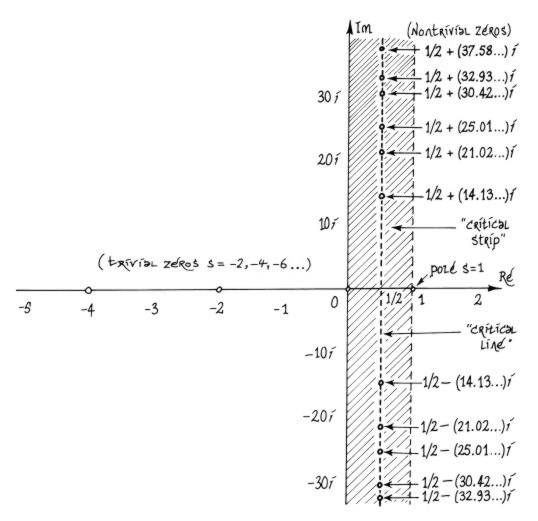The zeros of ζ(z), that is the points in the complex plane where ζ(z) = 0 , are of particular interest. They fall into two categories. The trivial zeros are the negative even integers –2, –4, –6, –8,..., so-called because it is relatively easy to demonstrate that ζ(2n) = 0 for natural numbers n. The nontrivial zeros are much more mysterious. Riemann was able to prove that they must all lie in the vertical strip defined by 0 < Re(z) < 1, which is now known as the critical strip. Further, due to the functional equation, which relates the values of ζ(z) and ζ(1–z), we are able to see that the set of all nontrivial zeros must be symmetric about both the real axis Im(z) = 0 and the critical line, the central axis of the critical strip defined by Re(z) = 1/2. That is, if a + bi is a nontrivial zero, then we know immediately that a – bi, (1–a) + bi and (1–a) – bi are also nontrivial zeros. Riemann was able to prove that there are an infinite number of nontrivial zeros. He computed the first few by hand and discovered that the all lay on the critical line. This led to the now-famous Riemann Hypothesis which states simply that all nontrivial zeros of ζ have real part 1/2. This has been confirmed for at least the first 1,500,000,000 zeros, using powerful computers, but this does not constitute a proof! Here are some tables of zeros compiled by Andrew Odlyzko. The proof of the Riemann Hypothesis has been called "the single most desirable achievement for a mathematician". << previous      beginning      next >>